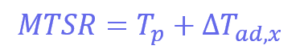## Process Development

#### Introduction

The key objective in Process Development is to define an appropriate basis of safety, identifying the conditions in which the reaction should be operated to minimize possible hazards and risks. It requires an understanding of the potential sources of thermal runaway risk, building on the preliminary screening studies carried out in Discovery, and understanding the process’s chemistry. This enables the process safety chemist to classify how dangerous the reaction is and what safe operating conditions are needed to mitigate the hazards identified.

It can be split into four main areas of investigation, the first three of which are illustrated in the reaction profile in Figure 4:

1. Desired reaction
2. Thermal runaway of reaction in the event of cooling failure
3. Decomposition/second thermal runaway?
4. How can the operating conditions be modified to reduce the risks identified?

### Understanding the desired reaction

Here, the key pieces of information to extract are:

• What is the energy evolved in the reaction?
• What is the cooling capacity required in the manufacturing plant to maintain an isothermal reaction?
• What is the subsequent rise in temperature when no cooling is applied?

Chemical reactions involve the transformation of reactants into products; heat will be exchanged with the environment in the process. In the case of exothermic reactions, energy will be released, and if the reaction is endothermic, energy will be absorbed. Understanding the thermodynamic nature of this transformation is fundamental. If endothermic and external energy is not supplied, the progression of the reaction can be very slow, making it inefficient. But on the other hand, if a chemical process is exothermic, associated risks might come, such as high temperature or high pressures.

Calorimeters can be used to perform the characterization of the chemical reaction. If the temperature is to be kept constant in the reaction (isothermal conditions), energy needs to be supplied to maintain this condition. This would tell us how much energy the reaction produces or takes. These energy values scale linearly, so we can calculate the energy required for the industrial scale using the value obtained from the calorimeter in a small-scale experiment.

As an example, we can use the esterification of acetic anhydride with methanol, which produces ethyl acetate and acetic acid:

(CH3CO)2O + CH3OH → CH3COOCH3 + CH3COOH

This is an exothermic reaction that releases 66 kJ mol-1. A calorimeter, like a Simular can be used. In this case, we can use a semi-batch regime, adding the reactant (acetic anhydride) at a feed rate of 2 g·min-1 over 50 mins and keeping the temperature at 30°C. As the reaction progresses, heat is released, and the cooling system activates to maintain a constant temperature. The figure below shows the power supplied to the reactor to keep the reaction isothermal (in W), how much acetic anhydride has been supplied (in g), and the energy released (in J).

In the following example of a semi-batch esterification reaction process, acetic anhydride was added at a 2 g/minute dosing rate over 50 minutes to methanol in a 1 L reactor in the Simular reaction calorimeter. At the same time, the temperature was held isothermally at 30°C . Figure 5 shows the:

• Reaction power (this is essentially the rate of heat generation) [pink trace]
• Energy released [blue trace]
• Feed rate [yellow trace]

Under these conditions, it can be seen that the reaction power reaches a maximum of 15W.  This means a cooling capacity of at least 15 W is required to keep this reaction isothermal.  As a first check, these values can be proportionately scaled up: for example, the reaction was performed in a 1 L reactor, so for a 2000 L pilot reactor, this would indicate that at least a 30 kW (30,000 W) cooling capacity is required for the larger system.

Also, the measured value for the energy released during the reaction is around 66 J, which progresses even when the reactor feed has finished. The energy released after the feed stopped is 34.6 kJ. This allows us to know the maximum temperature of synthesis reactions using the following equation:Where Tp is the temperature of the reactor (in this case, 30°C) and ∆𝑇𝑎𝑑,𝑥 is the adiabatic temperature increase. This is the change in the temperature when there is no heat exchange with the surroundings – so it just comes from the reaction itself. These values can be calculated using another equation:Where mCp is the thermal mass of the components (the mass multiplied by the heat capacity), and its value is 326 J°C-1. This means that the adiabatic temperature increase in the reactor is 106°C, so the total MTSR is 136°C. ∆𝑇𝑎𝑑,𝑥

Thus, reaction calorimetry enables the heat evolved during the desired reaction to be measured, which is then used to calculate the cooling capacity required to keep the system at the desired reaction temperature – Tp.

#### Solutions

The Simular measures the energy evolved in the reaction. Subsequently, this enables you to calculate the plant cooling capacity required to keep the reaction isothermal (Tp) and the maximum temperature the reaction can reach, helping predict the risks and reducing the hazards.  For this reason, the Simular can be used to explore and design safer reaction conditions, thereby facilitating the optimization of safe operations and minimizing process risk.

[Image_Link]

Simular | Process Development Reaction Calorimeter

This highly configurable system from the H.E.L product family excels in optimizing process...